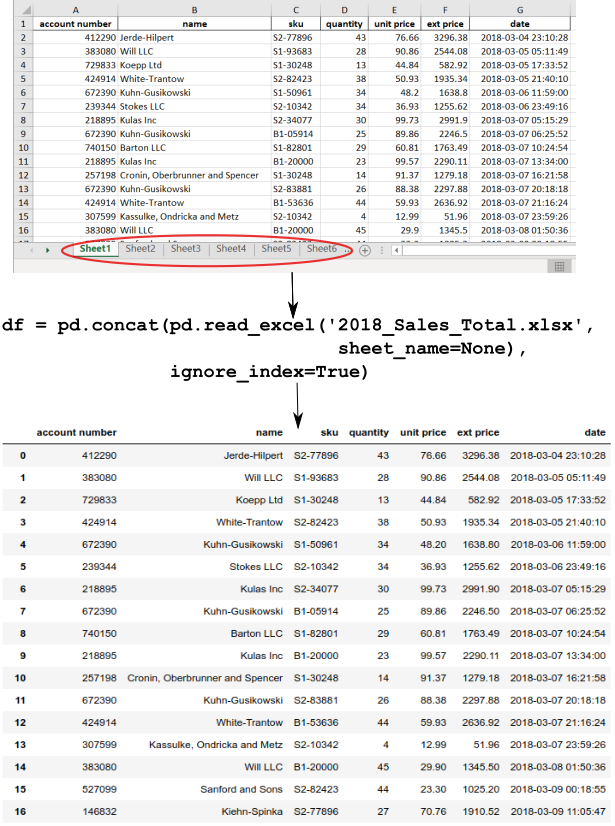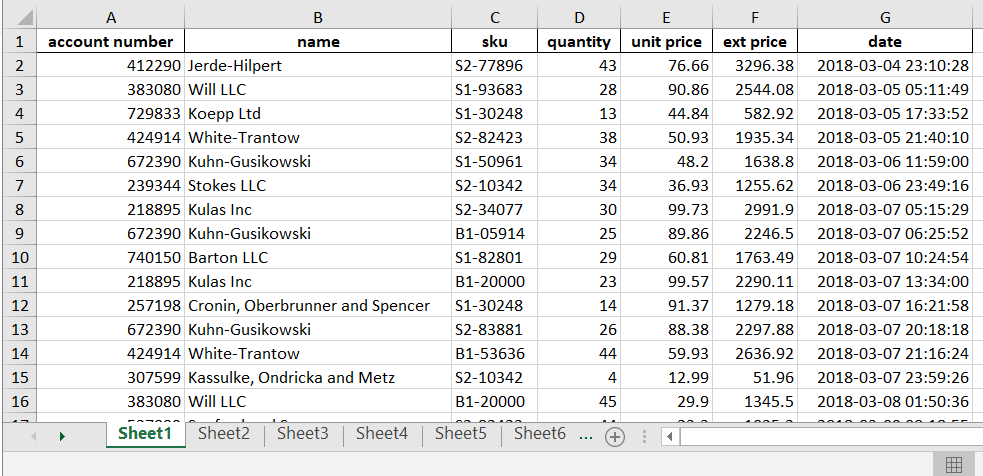Taking care of business, one python script at a time

Mon 26 August 2019

# Combine Multiple Excel Worksheets Into a Single Pandas Dataframe

Posted by Chris Moffitt in articles## Introduction

One of the most commonly used pandas functions is ``` read_excel``` . This short article shows how you can read in all the tabs in an Excel workbook and combine them into a single pandas dataframe using one command.

For those of you that want the TLDR, here is the command:

```df = pd.concat(pd.read_excel('2018_Sales_Total.xlsx', sheet_name=None), ignore_index=True)
```

Read on for an explanation of when to use this and how it works.

## Excel Worksheets

For the purposes of this example, we assume that the Excel workbook is structured like this:The process I will describe works when:

• The data is not duplicated across tabs (sheet1 is one full month and the subsequent sheets have only a single month’s worth of data)
• The columns are all named the same
• You wish to read in all tabs and combine them

The ``` read_excel``` function is a feature packed pandas function. For this specific case, we can use the ``` sheet_name``` parameter to streamline the reading in of all the sheets in our Excel file.

Most of the time, you will read in a specific sheet from an Excel file:

```import pandas as pd

workbook_url = 'https://github.com/chris1610/pbpython/raw/master/data/2018_Sales_Total_Tabs.xlsx'
```

If you carefully look at the documentation, you may notice that if you use ``` sheet_name=None``` , you can read in all the sheets in the workbook at one time. Let’s try it:

```all_dfs = pd.read_excel(workbook_url, sheet_name=None)
```

Pandas will read in all the sheets and return a ``` collections.OrderedDict``` object. For the purposes of the readability of this article, I’m defining the full url and passing it to ``` read_excel``` . In practice, you may decide to make this one command.

Let’s inspect the resulting ``` all_dfs``` :

```all_dfs.keys()
```
```odict_keys(['Sheet1', 'Sheet2', 'Sheet3', 'Sheet4', 'Sheet5', 'Sheet6'])
```

If you want to access a single sheet as a dataframe:

```all_dfs['Sheet1'].head()
```
account number name sku quantity unit price ext price date
0 412290 Jerde-Hilpert S2-77896 43 76.66 3296.38 2018-03-04 23:10:28
1 383080 Will LLC S1-93683 28 90.86 2544.08 2018-03-05 05:11:49
2 729833 Koepp Ltd S1-30248 13 44.84 582.92 2018-03-05 17:33:52
3 424914 White-Trantow S2-82423 38 50.93 1935.34 2018-03-05 21:40:10
4 672390 Kuhn-Gusikowski S1-50961 34 48.20 1638.80 2018-03-06 11:59:00

If we want to join all the individual dataframes into one single dataframe, use pd.concat:

```df = pd.concat(all_dfs, ignore_index=True)
```

In this case, we use ``` ignore_index``` since the automatically generated indices of ``` Sheet1``` , ``` Sheet2``` , etc. are not meaningful.

If your data meets the structure outlined above, this one liner will return a single pandas dataframe that combines the data in each Excel worksheet:

```df = pd.concat(pd.read_excel(workbook_url, sheet_name=None), ignore_index=True)
```

## Summary

This trick can be useful in the right circumstances. It also illustrates how much power there is in a pandas command that “just” reads in an Excel file. The full notebook is available on github if you would like to try it out for yourself.